2022: SklogWiki celebrates 15 years on-line

Equations of state for hard sphere mixtures

The following are equations of state for mixtures of hard spheres.

Mansoori, Carnahan, Starling, and Leland

The Mansoori, Carnahan, Starling, and Leland equation of state is given by (Ref. 1 Eq. 7):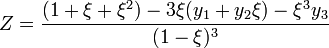$Z = \frac{(1+\xi + \xi^2)- 3\xi(y_1 + y_2 \xi) -\xi^3y_3 }{(1-\xi)^{3}}$

where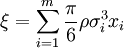$\xi = \sum_{i=1}^m \frac{\pi}{6} \rho \sigma_i^3 x_i$

where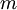$m$ is the number of components,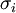$\sigma_i$ is the diameter of the$i$th component, and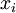$x_i$ is the mole fraction, such that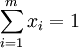$\sum_{i=1}^m x_i =1$.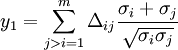$y_1 = \sum_{j>i=1}^m \Delta_{ij} \frac{\sigma_i + \sigma_j}{\sqrt{\sigma_i \sigma_j}}$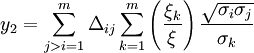$y_2 = \sum_{j>i=1}^m \Delta_{ij} \sum_{k=1}^m \left(\frac{\xi_k}{\xi} \right) \frac{\sqrt{\sigma_i \sigma_j}}{\sigma_k}$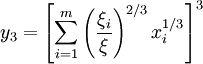$y_3 = \left[ \sum_{i=1}^m \left(\frac{\xi_i}{\xi} \right)^{2/3} x_i^{1/3} \right]^3$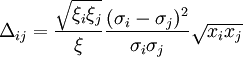$\Delta_{ij} = \frac{\sqrt{\xi_i \xi_j}}{\xi} \frac{(\sigma_i - \sigma_j)^2}{\sigma_i \sigma_j} \sqrt{x_i x_j}$

Ref. 2

Hansen-Goos and Roth

Ref. 3 Based on the Carnahan-Starling equation of state